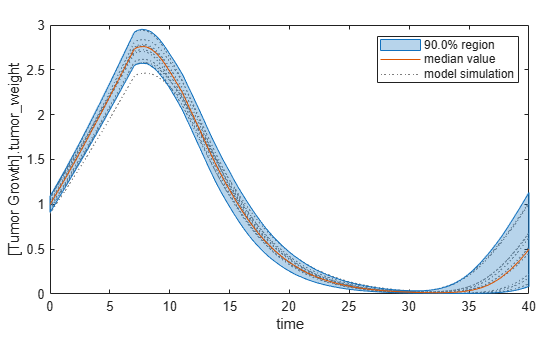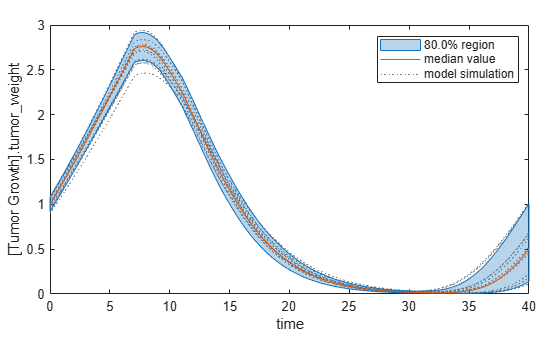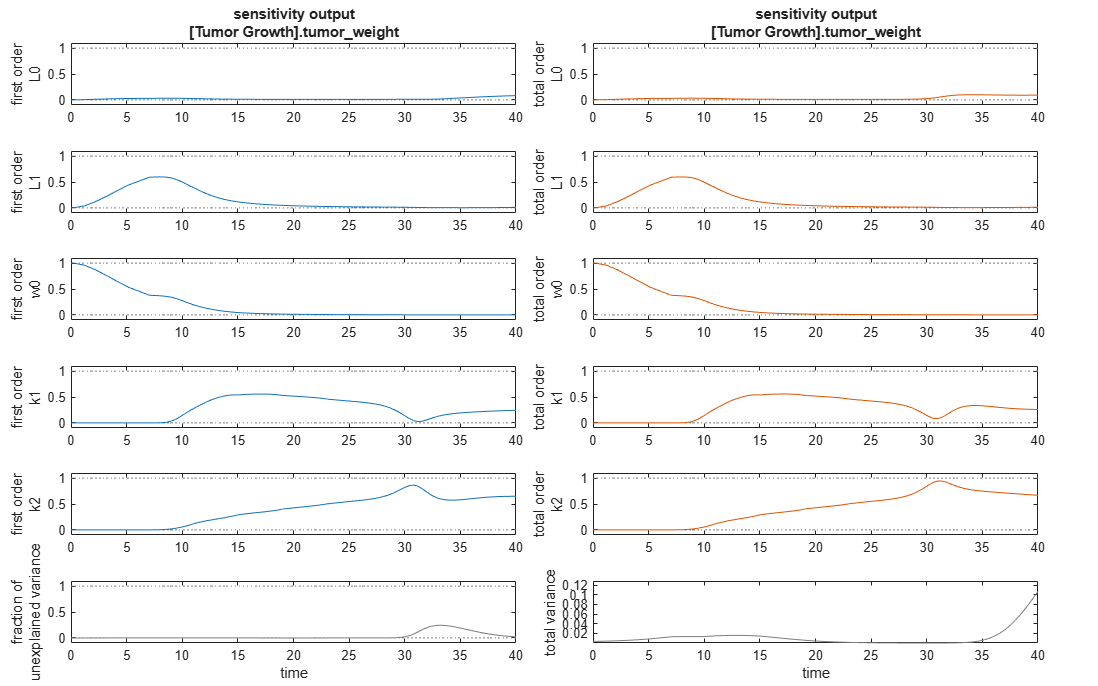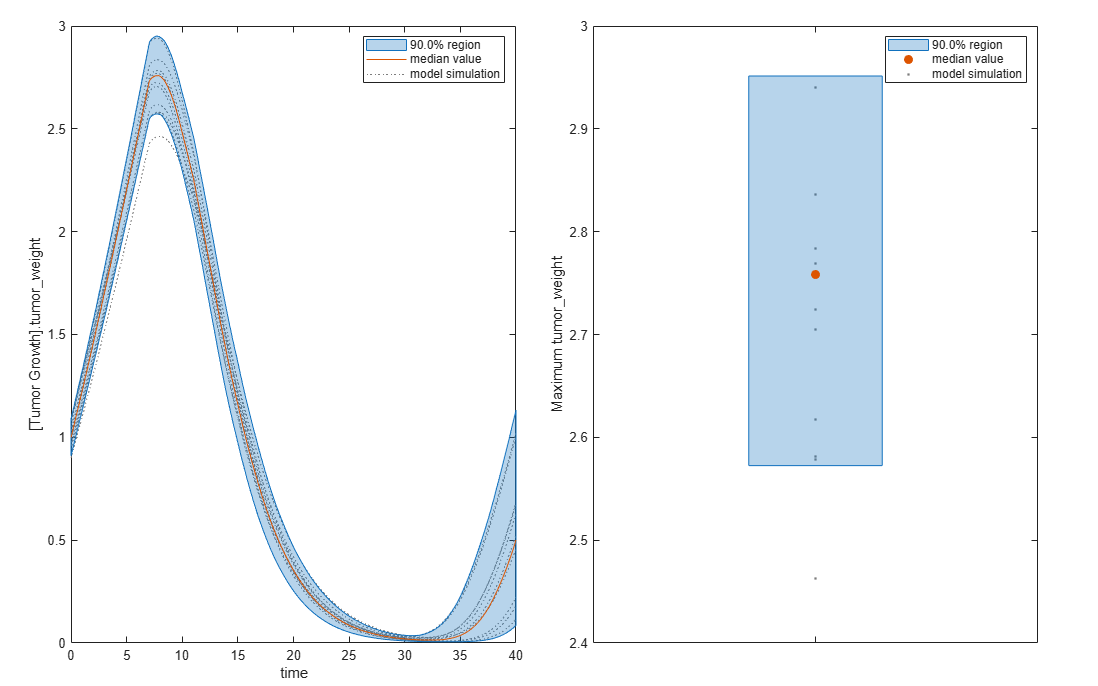# SimBiology.gsa.Sobol

Object containing first- and total-order Sobol indices

## Description

The `SimBiology.gsa.Sobol` object contains global sensitivity analysis results returned by `sbiosobol`. The object contains the computed first- and total-order Sobol indices related to the decomposition of the variance of model output with respect to sensitivity inputs .

## Creation

Create a `SimBiology.gsa.Sobol` object using `sbiosobol`.

## Properties

expand all

Sampled parameter values, specified as a table. The parameter sample values are used for approximating the Sobol indices. For details, see Saltelli Method to Compute Sobol Indices.

Data Types: `table`

Names of model responses or observables, specified as a cell array of character vectors.

Data Types: `char`

Time points at which Sobol indices are computed, specified as a column numeric vector. The property is `[]` if all observables are scalars.

Data Types: `double`

Computed sobol indices, specified as a structure array. The size of the array is `[params,observables]`, where params is the number of input parameters and observables is the number of observables.

Each structure contains the following fields.

• `Parameter` — Name of an input parameter, specified as a character vector

• `Observable` — Name of an observable, specified as a character vector

• `FirstOrder` — First-order Sobol index, specified as a numeric vector

• `TotalOrder` — Total-order Sobol index, specified as a numeric vector.

If all observables are scalar, then the `FirstOrder` and `TotalOrder` fields are specified as scalars. If some observables are scalars and some are vectors, `FirstOrder` and `TotalOrder` are numeric vectors of length `Time`. Scalar observables are scalar-expanded, where each time point has the same value.

Data Types: `struct`

Variance values for time courses of observables, specified as a table. Each column of the table contains the variance values for the time courses of each observable.

If all observables are scalars, then the `Variance` table has one row. If some observables are scalars and some are vectors, then the variances for scalar observables are scalar-expanded, where each row has the same value.

The `VariableNames` property of the table (`Variance.Properties.VariableNames`) is a cell array of character vectors containing the names of observables provided as inputs to `sbiosobol`. Names are truncated if needed. The `VariableDescriptions` property contains the untruncated observable names.

Data Types: `table`

Simulation information, such as simulation data and parameter samples, used for computing Sobol indices, specified as a structure. The structure contains the following fields.

• `SimFunction``SimFunction` object used for simulating model responses or observables.

• `SimData``SimData` array of size ```[NumberSamples,2 + params]```, where NumberSamples is the number of samples and params is the number of input parameters.

• The first column contains the model simulation results from `ParameterSamples`.

• The second column contains simulation results from `SupportSamples`.

• The rest of the columns contain simulation results from combinations of parameter values from `ParameterSamples` and `SupportSamples`. For information on retrieving the model simulation results and samples for a specified column (index) from this `SimData` array, see `getSimulationResults`. For details on how Sobol indices are computed, see the Saltelli Method to Compute Sobol Indices.

• `OutputTimes` — Numeric column vector containing the common time vector of all `SimData` objects.

• `Bounds` — Numeric matrix of size `[params,2]`. params is the number of input parameters. The first column contains the lower bounds and the second column contains the upper bounds for sensitivity inputs.

• `DoseTables` — Cell array of dose tables used for the `SimFunction` evaluation. `DoseTables` is the output of `getTable(doseInput)`, where doseInput is the value specified for the `'Doses'` name-value pair argument in the call to `sbiosobol` or `sbiompgsa`. If no doses are applied, this field is set to `[]`.

• `ValidSample` — Logical matrix of size ```[NumberSamples,2 + params]``` indicating whether a simulation result for a particular sample failed. Resampling of the simulation data (SimData) can result in `NaN` values if the data is extrapolated. Such SimData are indicated as invalid.

• `InterpolationMethod` — Name of the interpolation method for `SimData`.

• `SamplingMethod` — Name of the sampling method used to draw `ParameterSamples`.

• `RandomState` — Structure containing the state of `rng` before drawing `ParameterSamples`.

• `SupportSamples` — Table of parameter sample values used for approximating the Sobol indices. For details, see Saltelli Method to Compute Sobol Indices.

Data Types: `struct`

## Object Functions

 `resample` Resample Sobol indices to new time vector `addobservable` Compute Sobol indices for new observable expression `removeobservable` Remove Sobol indices of observables `getSimulationResults` Retrieve model simulation results and sample values used for computing Sobol indices `addsamples` Add additional samples to Sobol indices `plotData` Plot quantile summary of model simulations from global sensitivity analysis (requires Statistics and Machine Learning Toolbox) `plot` Plot first- and total-order Sobol indices and variances `bar` Create bar plot of first- and total-order Sobol indices

## Examples

collapse all

`sbioloadproject tumor_growth_vpop_sa.sbproj`

Get a variant with the estimated parameters and the dose to apply to the model.

```v = getvariant(m1); d = getdose(m1,'interval_dose');```

Get the active configset and set the tumor weight as the response.

```cs = getconfigset(m1); cs.RuntimeOptions.StatesToLog = 'tumor_weight';```

Simulate the model and plot the tumor growth profile.

`sbioplot(sbiosimulate(m1,cs,v,d));`Perform global sensitivity analysis (GSA) on the model to find the model parameters that the tumor growth is sensitive to.

First, retrieve model parameters of interest that are involved in the pharmacodynamics of the tumor growth. Define the model response as the tumor weight.

```modelParamNames = {'L0','L1','w0','k1','k2'}; outputName = 'tumor_weight';```

Then perform GSA by computing the first- and total-order Sobol indices using `sbiosobol`. Set `'ShowWaitBar'` to `true` to show the simulation progress. By default, the function uses 1000 parameter samples to compute the Sobol indices .

`sobolResults = sbiosobol(m1,modelParamNames,outputName,'Variants',v,'Doses',d,'ShowWaitBar',true)`
```sobolResults = Sobol with properties: Time: [444x1 double] SobolIndices: [5x1 struct] Variance: [444x1 table] Observables: {'[Tumor Growth Model].tumor_weight'} ParameterSamples: [1000x5 table] SimulationInfo: [1x1 struct] ```

You can change the number of samples by specifying the `'NumberSamples'` name-value pair argument. The function requires a total of `(number of input parameters + 2) * NumberSamples` model simulations.

Show the mean model response, the simulation results, and a shaded region covering 90% of the simulation results.

`plotData(sobolResults);`You can adjust the quantile region to a different percentage by specifying `'Alphas' `for the lower and upper quantiles of all model responses. For instance, an alpha value of 0.1 plots a shaded region between the `100 * alpha` and `100 * (1 - alpha)` quantiles of all simulated model responses.

`plotData(sobolResults,'Alphas',0.1);`Plot the time course of the first- and total-order Sobol indices.

```h = plot(sobolResults); % Resize the figure. h.Position(:) = [100 100 1280 800];```The first-order Sobol index of an input parameter gives the fraction of the overall response variance that can be attributed to variations in the input parameter alone. The total-order index gives the fraction of the overall response variance that can be attributed to any joint parameter variations that include variations of the input parameter.

From the Sobol indices plots, parameters `L1` and `w0` seem to be the most sensitive parameters to the tumor weight before the dose was applied at t = 7. But after the dose is applied, `k1` and `k2` become more sensitive parameters and contribute most to the after-dosing stage of the tumor weight. The total variance plot also shows a larger variance for the after-dose stage at t > 35 than for the before-dose stage of the tumor growth, indicating that `k1` and `k2` might be more important parameters to investigate further. The fraction of unexplained variance shows some variance at around t = 33, but the total variance plot shows little variance at t = 33, meaning the unexplained variance could be insignificant. The fraction of unexplained variance is calculated as 1 - (sum of all the first-order Sobol indices), and the total variance is calculated using `var(response)`, where `response` is the model response at every time point.

You can also display the magnitudes of the sensitivities in a bar plot.

`bar(sobolResults)`You can specify more samples to increase the accuracy of the Sobol indices, but the simulation can take longer to finish. Use `addsamples` to add more samples. For example, if you specify 1500 samples, the function performs `1500 * (2 + number of input parameters)` simulations.

```gsaMoreSamples = addsamples(gsaResults,1500) ```

The SimulationInfo property of the result object contains various information for computing the Sobol indices. For instance, the model simulation data (SimData) for each simulation using a set of parameter samples is stored in the `SimData` field of the property. This field is an array of `SimData` objects.

`sobolResults.SimulationInfo.SimData`
``` SimBiology SimData Array : 1000-by-7 Index: Name: ModelName: DataCount: 1 - Tumor Growth Model 1 2 - Tumor Growth Model 1 3 - Tumor Growth Model 1 ... 7000 - Tumor Growth Model 1 ```

You can find out if any model simulation failed during the computation by checking the `ValidSample` field of `SimulationInfo`. In this example, the field shows no failed simulation runs.

`all(sobolResults.SimulationInfo.ValidSample)`
```ans = 1x7 logical array 1 1 1 1 1 1 1 ```

`SimulationInfo.ValidSample` is a table of logical values. It has the same size as `SimulationInfo.SimData`. If `ValidSample` indicates that any simulations failed, you can get more information about those simulation runs and the samples used for those runs by extracting information from the corresponding column of `SimulationInfo.SimDat`a. Suppose that the fourth column contains one or more failed simulation runs. Get the simulation data and sample values used for that simulation using `getSimulationResults`.

`[samplesUsed,sd,validruns] = getSimulationResults(sobolResults,4);`

You can add custom expressions as observables and compute Sobol indices for the added observables. For example, you can compute the Sobol indices for the maximum tumor weight by defining a custom expression as follows.

```% Suppress an information warning that is issued during simulation. warnSettings = warning('off', 'SimBiology:sbservices:SB_DIMANALYSISNOTDONE_MATLABFCN_UCON'); % Add the observable expression. sobolObs = addobservable(sobolResults,'Maximum tumor_weight','max(tumor_weight)','Units','gram');```

Plot the computed simulation results showing the 90% quantile region.

```h2 = plotData(sobolObs); h2.Position(:) = [100 100 1280 800];```You can also remove the observable by specifying its name.

`gsaNoObs = removeobservable(sobolObs,'Maximum tumor_weight');`

Restore the warning settings.

`warning(warnSettings);`

expand all

## Tips

The results object can contain a significant amount of simulation data (SimData). The size of the object exceeds ```(1 + number of observables) * number of output time points * (2 + number of parameters) * number of samples * 8``` bytes. For example, if you have one observable, 500 output time points, 8 parameters, and 100,000 samples, the object size is `(1 + 1) * 500 * (2 + 8) * 100000 * 8` bytes = 8 GB. If you need to save such large objects, use this syntax:

`save(fileName,variableName,'-v7.3');`
For details, see MAT-file version.

 Saltelli, Andrea, Paola Annoni, Ivano Azzini, Francesca Campolongo, Marco Ratto, and Stefano Tarantola. “Variance Based Sensitivity Analysis of Model Output. Design and Estimator for the Total Sensitivity Index.” Computer Physics Communications 181, no. 2 (February 2010): 259–70. https://doi.org/10.1016/j.cpc.2009.09.018.

## SupportGet trial now